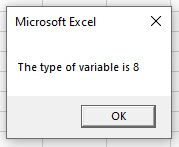# VBA VarType

This article will demonstrate the use of the VarType function in VBA.

VarType means Variable Type. We can supply the name of the variable to the VarType function, and it will return an integer which depicts the type of data stored in that variable according to a list of VBA constants.

## Using a Variant Variable to find the VarType

We can declare a variable as a Variant variable. This will allow us to store any type of allowed data in it. We can then populate this variable, and use the VarType function to see what type of data is in the variable.

For example:

``````Sub TestVarType()
Dim MyVariable As Variant
MyVariable = "Hello World"
MsgBox "The type of variable is " & VarType(MyVariable)
End Sub``````

This will return the following message box:The value of 8 is therefore returned.  This in an Excel Constant which defines the type of data that is stored in the variable.

This constant value corresponds to the table below:

Constant Value Description
vbEmpty 0 Empty (uninitialized)
vbNull 1 Null (no valid data)
vbInteger 2 Integer
vbLong 3 Long integer
vbSingle 4 Single-precision floating-point number
vbDouble 5 Double-precision floating-point number
vbCurrency 6 Currency value
vbDate 7 Date value
vbString 8 String
vbObject 9 Object
vbError 10 Error value
vbBoolean 11 Boolean value
vbVariant 12 Variant (used only with arrays of variants)
vbDataObject 13 A data access object
vbDecimal 14 Decimal value
vbByte 17 Byte value
vbLongLong 20 LongLong integer (valid on 64-bit platforms only)
vbUserDefinedType 36 Variants that contain user-defined types
vbArray 8192 Array (always added to another constant when returned by this function)

We can try another example:

``````Sub TestVarType()
Dim MyVariable As Variant
MyVariable = 32760
MsgBox "The type of variable is " & VarType(MyVariable)
End Sub``````

This time the message box will return a 2 as the type of data that is stored in the variable is Integer.

However, if we change the value to 32780, then the value return will be 3 – Long Integer as an Integer data type has a maximum value of 32767; after which it is a Long Integer.

If we were to once again amend the code:

``````Sub TestVarType()
Dim MyVariable As Variant
MyVariable = 32760.52
MsgBox "The type of variable is " & VarType(MyVariable)
End Sub``````

We would now  get a value of 5 being returned – the data type is Double due to the decimal places.

If we were to put the value in the variable in quotes, then once again an 8 would be returned as any value contained within quotes is stored as a string value.

``````Sub TestVarType()
Dim MyVariable As Variant
MyVariable = "32760.52"
MsgBox "The type of variable is " & VarType(MyVariable)
End Sub``````

Stop searching for VBA code online. Learn more about AutoMacro - A VBA Code Builder that allows beginners to code procedures from scratch with minimal coding knowledge and with many time-saving features for all users!